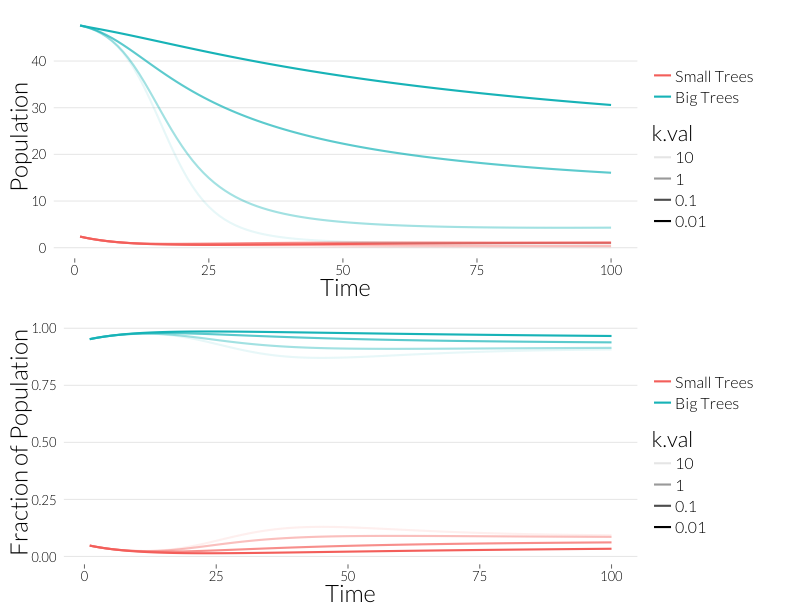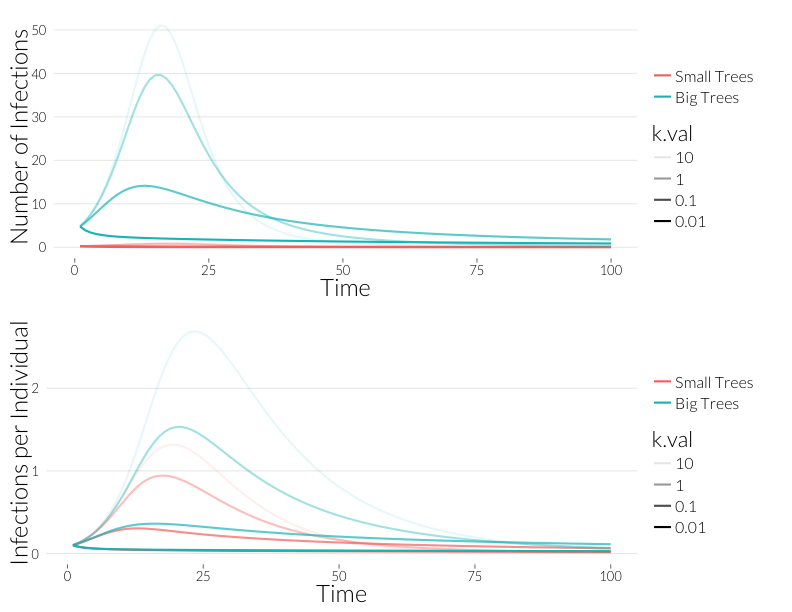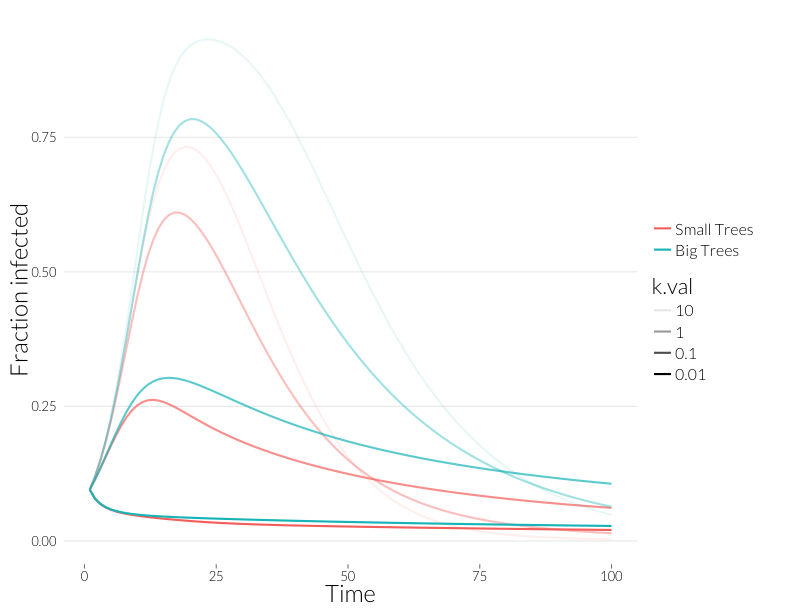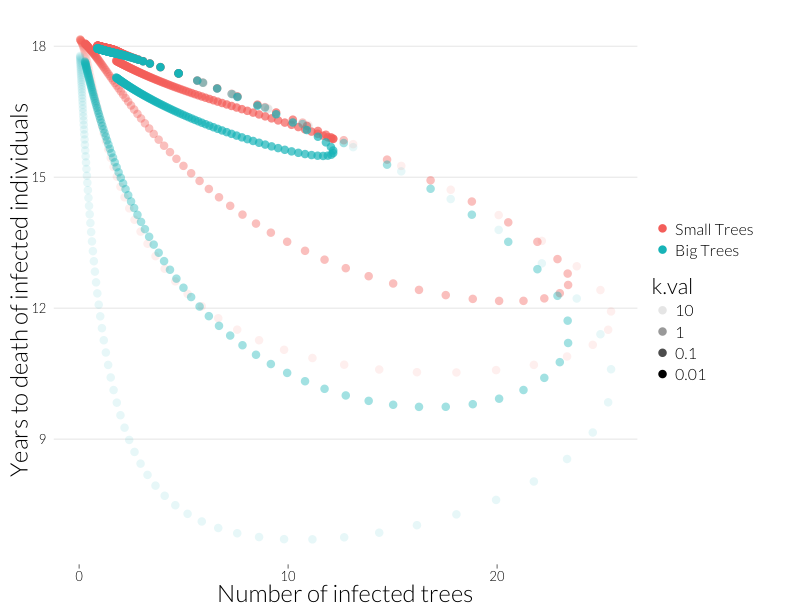# The null model for age effects with overdispersed infection

#### June 12, 2013

How does overdispersion of infections affect the behavior of the multiple-infection model? I redefine the model to account for overdispersion, assuming the same overdispersion occurs in both age classes. The parameter $$k$$ varies inversely with the degree of overdispersion. Again, the classes are demographically identical, and infection affects mortality but not growth:

\begin{aligned} \frac{dJ}{dt} &= A f_A \left(1 - \frac{J+A}{K} \right) + J \left(f_J \left(1 - \frac{J+A}{K} \right) - d_J - g\right) - \alpha P_J \\ \frac{dA}{dt} &= J g - A d_A - \alpha P_A \\ \frac{dP_J}{dt} &= \lambda \frac{J}{K} (P_J + P_A) - P_J \left(d_J + \mu + g + \alpha \left(1 + \frac{(k+1)P_J}{kJ} \right) \right) \\ \frac{dP_A}{dt} &= \lambda \frac{J}{K} (P_J + P_A) + P_J g - PA \left(d_A + \mu + \alpha \left(1 + \frac{(k+1)P_A}{kA} \right) \right) \end{aligned}

I define the model and run it in R below, using values of $$k$$ ranging from 0.01 to 10. Otherwise all parameters and conditions are the same as in my last post.

od.model <- function(t, y, parms) {
list2env(as.list(y), environment())
list2env(as.list(parms), environment())
dJ <- A*f_a*(1 - (J+A)/K) + J * (f_j*(1 - (J+A)/K) - d_j - g) - alpha * PJ
dA <- J*g - (A * d_a) - alpha * PA
dPJ <- lambda * (PJ + PA) * J/K -
PJ*(d_j + mu + g + alpha*(1 + (k+1)*PJ/(k*J)))
dPA <- lambda * (PJ + PA) * A/K + PJ * g -
PA*(d_a + mu + alpha*(1 + (k+1)*PA/(k*A)))
return(list(c(dJ=dJ, dA=dA, dPJ=dPJ, dPA=dPA),
c(dJ=dJ, dA=dA, dPJ=dPJ, dPA=dPA)))
}

parms <- c(
f_j=0.01,
f_a=0.01,
g=0.1,
d_j=0.005,
d_a=0.005,
alpha=0.05,
lambda=0.3,
K=50,
mu=0.00,
k=0.000
)

A_ss = with(as.list(parms), K/(d_a/g + 1))
J_ss = with(as.list(parms), K - A_ss)
init <- c(J=J_ss, A=A_ss, PJ=0.1*J_ss, PA=0.1*A_ss)

require(deSolve)
require(reshape2)
require(plyr)
ks <- c(10, 1, 0.1, 0.01)
df <- adply(ks, 1, function(x) {
parms["k"] <- x
df <- data.frame(k.val=as.factor(x),
as.data.frame(lsoda(y=init, times=1:100, func=od.model,
parms=parms)))
})

names(df)[names(df)=="time"] <- "Time"
df\$X1 <- NULL

df <- within(df, {
list2env(as.list(parms), environment())
pctJ <- J/(J + A)
pctA <- A/(J + A)
nJ <- PJ / J
nA <- PA / A
J.inf <- 1 - exp(-nJ)
A.inf <- 1 - exp(-nA)
Inf.dens <- (J*J.inf + A*A.inf)
J.mort <- d_j + alpha * PJ / (J *J.inf)
A.mort <- d_a + alpha * PA / (A * A.inf)
J.yrs <- 1/J.mort
A.yrs <- 1/A.mort
J.infrate <- 1 - exp(-lambda * (PJ + PA) * J * exp(-PJ/J) / K)
A.infrate <- 1 - exp(-lambda * (PJ + PA) * A * exp(-PA/A) / K)
J.infyrs <- 1/J.infrate
A.infyrs <- 1/A.infrate
rm(list=names(parms))
})

df <- df[,sort(names(df), decreasing=TRUE)]

mdf <- melt(df, id.vars=c("Time", "Inf.dens", "k.val"), variable.name="Class",
value.name="Population")

Now I plot the results. In all of the following the darker lines represent the case with more overdispersion, and the lighter case are closer to random distribution of infections.

require(ggplot2)
require(gridExtra)
theme_nr <- theme_nr + theme(legend.title=element_text(size=22),
legend.text=element_text(size=16),
legend.key.size=unit(.75, "cm"))
JAlab <- scale_color_discrete(labels=c("Small Trees","Big Trees"), name="")
p1 <- ggplot(subset(mdf, Class %in% c("J", "A")),
aes(x=Time, y=Population, col=Class, alpha=k.val)) +
geom_line(lwd=1) + theme_nr + ylab("Population") + JAlab
p2 <- ggplot(subset(mdf, Class %in% c("pctJ", "pctA")),
aes(x=Time, y=Population, col=Class, alpha=k.val)) +
geom_line(lwd=1) + theme_nr + ylab("Fraction of Population") + JAlab
grid.arrange(p1, p2, nrow=2)In general, overdispersion appears to reduce the decline of the population. This is because fewer, more heavily infected individuals carry more of the infections in the population, and take those away when they die. I wonder how biologically realistic is this in the case of SOD? Now I look at infection rates:

p3 <- ggplot(subset(mdf, Class %in% c("PJ", "PA")),
aes(x=Time, y=Population, col=Class, alpha=k.val)) +
geom_line(lwd=1) + theme_nr + ylab("Number of Infections") + JAlab
p4 <- ggplot(subset(mdf, Class %in% c("nJ", "nA")),
aes(x=Time, y=Population, col=Class, alpha=k.val)) +
geom_line(lwd=1) + theme_nr + ylab("Infections per Individual") + JAlab
grid.arrange(p3, p4, nrow=2)As you can see, the overall infections rate are lower in the case of overdispersion.

ggplot(subset(mdf, Class %in% c("J.inf", "A.inf")),
aes(x=Time, y=Population, col=Class, alpha=k.val)) +
geom_line(lwd=1) + theme_nr + ylab("Fraction infected") + JAlabThe fraction of the population infected when there is some overdispersion is more in line with what is seen in the field in many cases.

ggplot(subset(mdf, Class %in% c("J.yrs", "A.yrs") ),
aes(x=Inf.dens, y=Population, col=Class, alpha=k.val)) +
geom_point(cex=4) + theme_nr + xlab("Number of infected trees") +
ylab("Years to death of infected individuals") + JAlabWith less infection, there’s less of a dramatic difference between the upswing and downswing of the disease, but the relationship between infected trees and years-to-death still holds.

In general, overdispersion seems to dampen the effects of infection, but most qualitative patterns remain.

In some ways, overdispersal has a similar effect to space in the Cobb et al. (2012) $$SIR$$ model. Spatial structure, of course, is one mechanism that can create overdispersal.

Cobb, R. C., J. A. N. Filipe, R. K. Meentemeyer, C. A. Gilligan, and D. M. Rizzo. 2012. Ecosystem transformation by emerging infectious disease: loss of large tanoak from California forests. Journal of Ecology 100:712–722.

← A Null Model for Age Effects in Disease with Multiple Infections | All posts | Dave Harris on Maximum Likelihood Estimation →